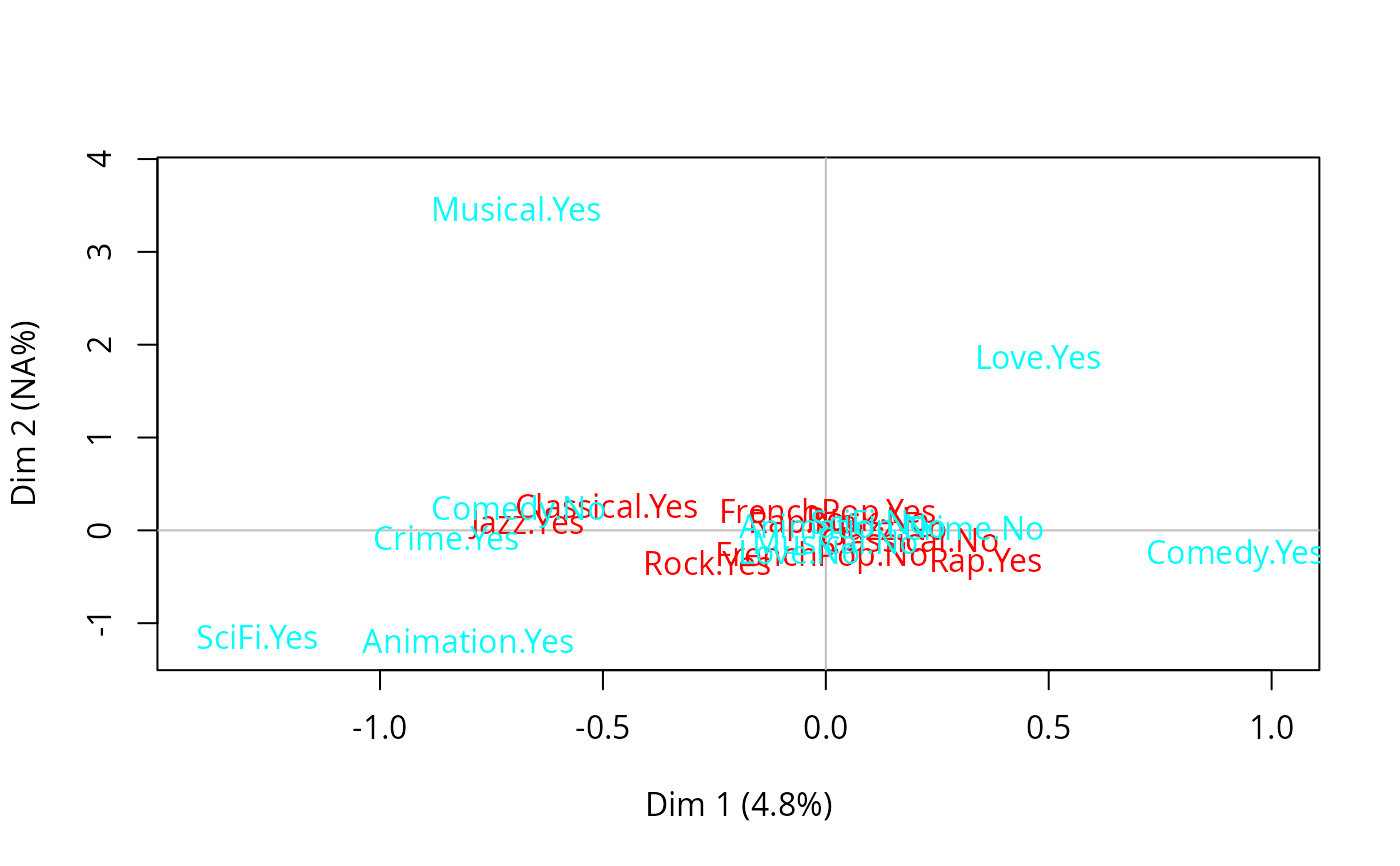Performs Multiple Factor Analysis, drawing on the work of Escofier and Pages (1994). It allows the use of MCA variants (e.g. specific MCA or class specific MCA) as inputs.

multiMCA(l_mca, ncp = 5, compute.rv = FALSE)

## Arguments

l_mca

a list of objects of class MCA, speMCA or csMCA

ncp

number of dimensions kept in the results (default is 5)

compute.rv

whether RV coefficients should be computed or not (default is FALSE, which makes the function execute faster)

## Details

This function binds individual coordinates from every MCA in l_mca argument, weights them by the first eigenvalue, and the resulting data frame is used as input for Principal Component Analysis (PCA).

## Value

Returns an object of class multiMCA, i.e. a list:

eig

a list of numeric vector for eigenvalues, percentage of variance and cumulative percentage of variance

var

a list of matrices with results for input MCAs components (coordinates, correlations between variables and axes, squared cosines, contributions)

ind

a list of matrices with results for individuals (coordinates, squared cosines, contributions)

call

a list with informations about input data

VAR

a list of matrices with results for categories and variables in the input MCAs (coordinates, squared cosines, test-values, variances)

my.mca

lists the content of the objects in l_mca argument

RV

a matrix of RV coefficients

## Author

Nicolas Robette

plot.multiMCA, speMCA, csMCA

## Examples

data(Taste)
# specific MCA on music variables of Taste example data set
mca1 <- speMCA(Taste[,1:5], excl = c(3,6,9,12,15))
# specific MCA on movie variables of Taste example data set
mca2 <- speMCA(Taste[,6:11], excl = c(3,6,9,12,15,18))
# Multiple Factor Analysis of the two sets of variables
mfa <- multiMCA(list(mca1,mca2))
plot.multiMCA(mfa)